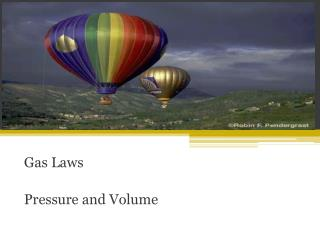DownloadDownload PresentationGas Laws Pressure and Volume

# Gas Laws Pressure and Volume

Télécharger la présentation## Gas Laws Pressure and Volume

- - - - - - - - - - - - - - - - - - - - - - - - - - - E N D - - - - - - - - - - - - - - - - - - - - - - - - - - -
##### Presentation Transcript

1. Gas Laws Pressure and Volume

2. Bell Work Three soda cans are placed into three different situations as shown below. Which soda can has particles with the highest kinetic energy and why? What does this do to the pressure inside the can?

3. Presentations about Pressure and Volume

4. Pressure and Volume • Increase volume , decrease pressure • Decrease volume, increase pressure • Pressure and volume are inversely related • Cut volume in half and pressure doubles

5. Combined Gas Law • P = pressure • V = volume • n= moles • T = temperature • Subscript 1 = initial • Subscript 2 = final

6. Read the problem • What variables are we dealing with? • Cover (Cancel) out extra variables • Write down variables and givens • Plug-in and Solve A gas is collected in a 242 mL container. The pressure of the gas in the container is measured and determined to be 87.6 kPa. What is the volume of this gas at 101.3 kPa? Assume the temperature is constant. P2= 101.3 kPa V2= ? P1 = 87.6 kPa V1= 242 mL

7. What is STP?

8. Read the problem • What variables are we dealing with? • Cover (Cancel) out extra variables • Write down variables and givens • Plug-in and Solve If 1.0 L of a gas at standard temperature and pressure is compressed to 0.473 L, what is the new pressure of the gas? P2= ? V2= 0.473 L P1 = 1.00 atm V1= 1.0 L